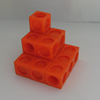#### You may also like### Picture Story

Can you see how this picture illustrates the formula for the sum of the first six cube numbers?### The Root of the Problem

Find the sum of this series of surds.### Sums of Powers - A Festive Story

A story for students about adding powers of integers - with a festive twist.

# Slick Summing

### Why do this problem?

This problem provides an introduction to summing simple arithmetic series. By considering a particular case, students are invited to attend to the structure and see where the general method for summing such series comes from.

The Stage 5 problem Speedy Summations poses more challenging questions using $\sum$ notation.

### Possible approach

This printable worksheet may be useful: Slick Summing.

You may wish to show the video, in which Charlie works out $1+2+3+4+5+6+7+8+9+10$ in silence, or you may wish to recreate the video for yourself on the board.

"With your partner, discuss what you saw. Can you recreate the video for yourselves? Can you explain what happened?"

Once students have had a chance to make sense of the video:

"I wonder if you could adapt Charlie's method to work out some similar questions?

Try $1 + 2 + 3 + \dots + 19 + 20$ first."

While students are working, write up a few more questions on the board, such as

$1 + 2 + 3 + \dots + 99 + 100$
and
$40 + 41 + 42 + \dots + 99 + 100$

Circulate to check for misconceptions - one common one is that the sum from 1 to 20 will be twice the sum from 1 to 10, and for the last example above, some students may assume the sequence has 60 terms.

Bring the class together to discuss what they have done.

"I wonder whether Charlie's method could be adapted for sequences that don't go up in ones?"

$1 + 3 + 5 + \dots + 17 + 19$
$2 + 4 + 6 + \dots + 18 + 20$
$42 + 44 + 46 + \dots + 98 + 100$

"In a while I'm going to give you another question like these and you'll need to have developed a strategy to work it out efficiently."

While students are working, listen out for useful comments that they make about how to work out such sums generally. Then bring the class together to share answers and methods for the questions they have worked on.

Make up a few questions like those above, and invite students out to the board to work them out 'on the spot', explaining what they do as they go along. This might be an opportunity to invite more than one student to the board at the same time to work on the same question.

Next, invite students to create a formula from their general thinking:
"Imagine summing the sequence $1 + 2 + \dots + (n-1) + n$.

Can you use what you did with the numerical examples to create an expression for this sum?"

Give students time to think and discuss in pairs and then build up the formula together.

### Key questions

What can you say about the sum of the first and last, and the second and penultimate terms of these sequences?

How do you know these sums of pairs will always be the same?

### Possible support

Picturing Triangle Numbers offers a pictorial representation of the sum of the first $n$ integers

### Possible extension

Speedy Summations contains some challenging follow-up questions.